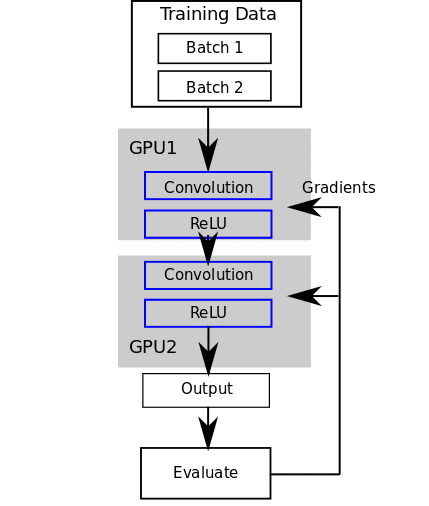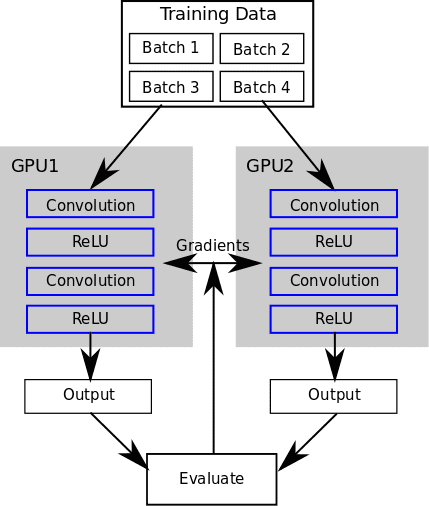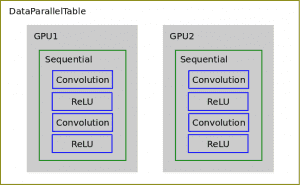Deep Learning with Multiple GPUs on Rescale: TorchToday we will discuss how to make use of multiple GPUs to train a single neural network using the Torch machine learning library. This is the first in a series of articles on techniques for scaling up deep neural network (DNN) training workloads to use multiple GPUs and multiple nodes.
In this series, we will be focusing on parallelizing the training of a single network. For more about the embarrassingly parallel problem of training multiple networks efficiently to optimize configuration parameters, see this earlier post on hyper-parameter optimization.
Torch is a lightweight, flexible tensor library built on top of the Lua programming language. Torch is popular with machine learning researchers, so many new deep neural network ideas are first implemented in Torch and made available as open source extensions. Thus, the state-of-the-art in deep learning is often first available to use in Torch.
The downside of this is that Torch documentation often falls behind implementation, so unless you find an example on github for exactly what you want to do, it can be a challenge to figure out which Torch modules you should be using and how to use them.
One example of this is how to get Torch to train your neural networks using multiple GPUs. Searching for “multi gpu torch” on the internet yields this github issue as one of the top results. From this, we know we can access more than one GPU from the torch environment, but how do we use this low-level construct to train a complex network?
Data vs. Model Parallelism
When parallelizing the work to train a single neural network, we have 2 choices on how to split up the work: Model Parallelism and Data Parallelism.With Model Parallelism, each GPU runs a chunk of the nodes in the network for a given batch of data.With Data Parallelism, each GPU runs the entire network for different batches of data.
This distinction is discussed in detail in this paper, but the choice between using one or the other impacts what kind of synchronization is required between GPUs. Data parallelism requires synchronization of model parameters, model parallelism requires synchronizing input and output values between the chunks.
Simple Torch Example
We will now look at a simple example of training a convolutional neural network based on a unit test in Torch itself. This network has 2 convolutions layers and 2 rectifier layers. We do a simple forward and backward pass over the network. Instead of actually computing error gradients for training, we just set them to a random vector to keep things simple.

require 'nn'
model = nn.Sequential()
input = torch.round(torch.Tensor(16, 3, 10, 10):uniform(0, 255))
output = model:forward(input)

Now let’s convert it to run on a GPU (this example will only run if you have a CUDA-compatible GPU):

require 'cutorch'
require 'cunn'
cutorch.setDevice(1)
model = nn.Sequential()
model:cuda()
input = torch.round(torch.CudaTensor(16, 3, 10, 10):uniform(0, 255))
output = model:forward(input)

To run this on a GPU, we call cuda()on the network and then make the input a CudaTensor.
Now let’s distribute the model across 2 GPUs (as an example of the model parallel paradigm). We iterate over the GPU device IDs and use the cutorch.withDevice to place each layer on a particular GPU.

require 'cutorch'
require 'cunn'
cutorch.setDevice(1)
model = nn.Sequential()
for i=1, math.min(2, cutorch.getDeviceCount()) do
cutorch.withDevice(i, function() model:add(nn.SpatialConvolution(3, 3, 3, 5)) end)
end
model:cuda()
input = torch.round(torch.CudaTensor(16, 3, 10, 10):uniform(0, 255))
output = model:forward(input)

This puts a convolutional layer and a ReLU layer on each GPU. The forward and backward passes must propagate the outputs between GPU 1 and GPU 2.
Next, we use nn.DataParallelTable to distribute batches of data to copies of the whole network running on multiple GPUs. DataParallelTable is a Torch Container that wraps multiple Containers and distributes the input across them.require 'cutorch'
require 'cunn'
cutorch.setDevice(1)
model = nn.Sequential()
model:cuda()
gpus = torch.range(1, cutorch.getDeviceCount()):totable()
input = torch.round(torch.CudaTensor(16, 3, 10, 10):uniform(0, 255))
output = dpt:forward(input)

So instead of running the forward and backward passes over the original Sequential container, we now run it on the DataParallelTable container and the data is distributed to copies of the network on each GPU.
Here is a job on Rescale you can clone and run yourself with all the above code.
A Larger Example
Let’s now look at a use of DataParallelTable in action when training a real DNN. We will be using Sergey Zagoruyko’s implementation of Wide Residual Networks on CIFAR10 on github.
In train.lua, we see all the parallelization of the base neural network is applied by a helper function:

Delving into makeDataParallelTable, we see a similar structure to our last example above using nn.DataParallelTable:add

function utils.makeDataParallelTable(model, nGPU)
if nGPU > 1 then
local gpus = torch.range(1, nGPU):totable()
local fastest, benchmark = cudnn.fastest, cudnn.benchmark
local dpt = nn.DataParallelTable(1, true, true)
local cudnn = require 'cudnn'
cudnn.fastest, cudnn.benchmark = fastest, benchmark
end)